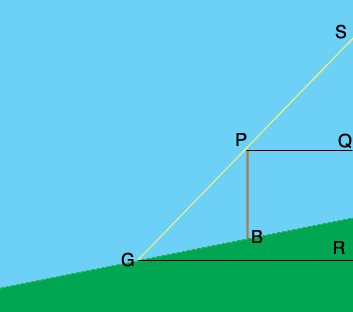SEARCH HOMEMath Central Quandaries & QueriesQuestion from Marissa, a student: A 10 meter telephone pole casts a 17 meter shadow directly down a slope when the angle of elevation of the sun is 42 degrees. Find the angle of elevation of the ground. Its a law of sines problem.Hi Marissa,PB is the pole and PP is the sun ray. I think you are to assume the pole is vertical. The lines PQ and GR are horizontal and hence the angle SPQ is the angle of elevation of the sum which you are told is 42o. What is the measure of the angle BPG?

You know the lengths of GB and BP so the Law of Sines gives you the measure of the angle PGB. What is the measure of the angle BGR? This is the angle of elevation of the ground.

I hope this helps,
PennyMath Central is supported by the University of Regina and The Pacific Institute for the Mathematical Sciences.